# Block convoluiton using skew transform (overlap-save method)

## Overlap-save

```function []=overlapsaveqdft_demo()
```
```% Classic overlapsave method is using FFT size of 2N, where N is block size.
% Proposed method is using skew transform of size N for computation reduction.
% Convolution mod(x^N-j) is used for each block, and the skew transform is an eigen transform of this skew convolution.
% It is easy to see that multiplication of two polynomials y(z)=x(z)*h(z) (mod (x^N-j)), where x(z)=(x(0)+j*x(N))+(x(1)+j*x(N+1))*z+(x(2)+j*x(N+2))*z^2+..+(x(N-1)+j*x(2*N-1))*z^(N-1) is
% combined of two adjacent blocks of input signal,
% and h(z)=h(0)+h(1)*z+h(2)*z^2+..+h(N-1)*z^(N-1) is impulse response of a filter, will give convolution result for the block if output
% is read as Y=[Re(y(0))... Re(y(N-1))].
```

## Init

```N=32;   % block size

x=randn(1,5*N);     % input signal
h=randn(1,N);       % filter impulse response

% y=fftfilt(h,x);   % true result
```

## Classic overlap-save method (FFT size = 2*N)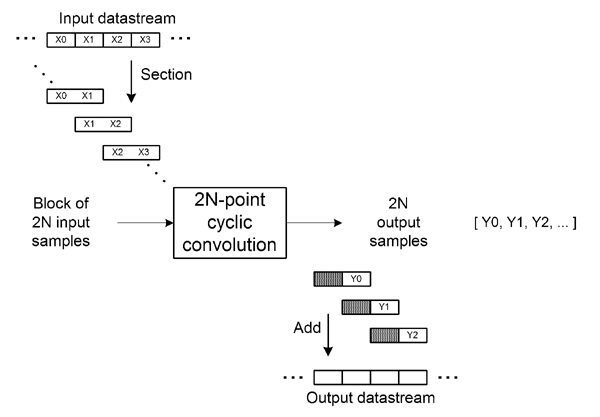```hs=fft(h',2*N);                 % filter spectral response
xn=reshape(x,N,[]);
xn=[zeros(N,1) xn(:,1:end-1); xn];
% xn=[xn; zeros(size(xn))];
xs=fft(xn);                     % block spectrum of input signal (FFT size = 2*N)
ys=bsxfun(@times,hs,xs);        % spectral domain multiplication
y1=real(ifft(ys));              % block convolution result
ycl=reshape(y1((1:N)+N,:),[],1);

% block-wise graph
figure(2)
plot_blockwise_classic(y1,N,length(x))

subplot(size(y1,2)+1,1,size(y1,2)+1);
plot(ycl)
axis([0 round(length(x)+N*1.1) -20 20]);
grid on
ylabel(['output']);
```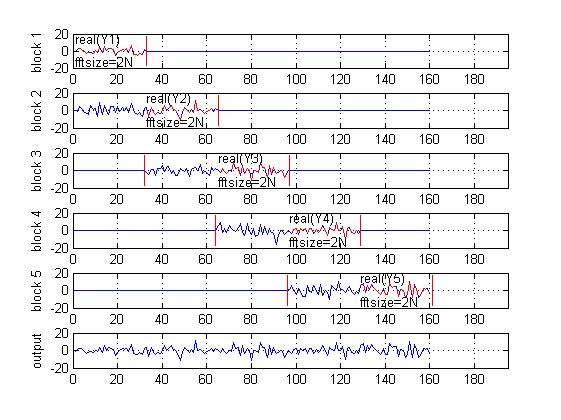## Proposed overlap-save method (FFT size = N)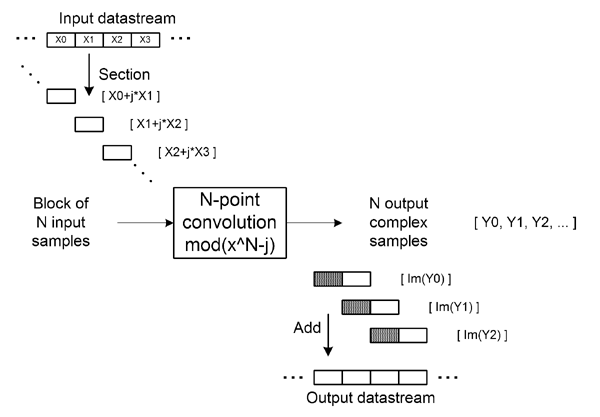```w=exp(1i*pi/2/N*(0:N-1)');         % diagonal elements for qDFT
hs=fft(w.*h');

xn=reshape(x,N,[]);
xn(:,2:end)=xn(:,1:end-1)+1i*xn(:,2:end); xn(:,1)=1i*xn(:,1); % combining input blocks
x1=bsxfun(@times,w,xn);                 % pre-multiplication by diagonal twiddle factors
s1=fft(x1);                             % block spectrum of input signal (qDFT size = N)
s2=bsxfun(@times,hs,s1);                % spectral domain multiplication
y1=bsxfun(@times,conj(w),ifft(s2));     % block convolution result
ypr=reshape(imag(y1),1,[]);             % overlap-save combination

% block-wise graph
figure(1)
% title('Proposed qDFT based method');
plot_blockwise_propose(y1,N,length(x))

% plot resulting output
subplot(size(y1,2)+1,1,size(y1,2)+1);
plot(ypr)
axis([0 round(length(x)+N*1.1) -20 20]);
grid on
ylabel(['output']);
```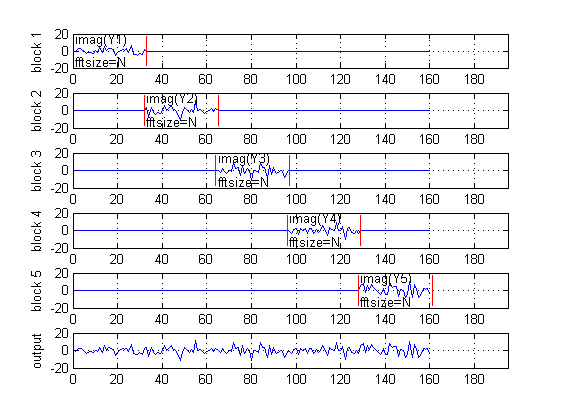## Compare results

```figure(3)
plot(ycl)
hold on
plot(ypr,'--r');
hold off
grid on
legend('classic','proposed')
```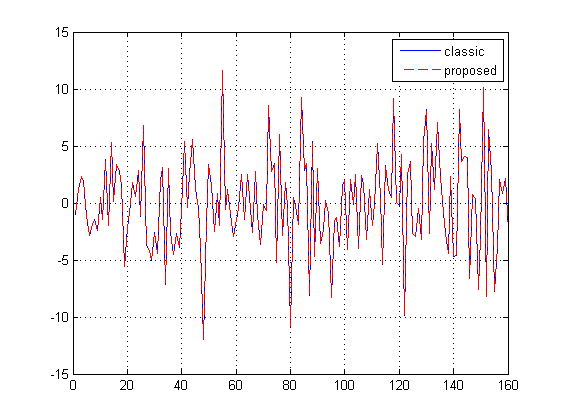## References

 Blahut, R.E.: Fast Algorithms for Signal Processing. Cambridge University Press (2010). (p. 198)  Jung Gap Kuk, Seyun Kim, and Nam Ik Cho, "A New Overlap Save Algorithm for Fast Block Convolution and Its Implementation Using FFT," Journal of Signal Processing Systems(Online), Feb 2010.

```function []=plot_blockwise_classic(y1,N,lenx)
for i=1:size(y1,2),
y9=zeros(size(y1));
y9(:,i)=y1(:,i);
yt=y9((1:N)+N,i);
xt=(i-1)*N+(1:N);
y9=reshape(y9((1:N)+N,:)+[y9(1:N,2:end) zeros(N,1)],1,[]);
subplot(size(y1,2)+1,1,i);
plot(y9)
hold on
plot(xt,yt,'--r');
a=17;
plot([0 0]+(i-1-1)*N,[-a a],'r');
plot([1 1]+(i-1+1)*N,[-a a],'r');
text(1+(i-1)*N,-a*0.8,'fftsize=2N');
text(1+(i-1)*N,a*0.8,['real(Y' num2str(i) ')']);
hold off
axis([0 round(lenx+N*1.1) -20 20]);
grid on
ylabel(['block ' num2str(i)]);
end

function []=plot_blockwise_propose(y1,N,lenx)
for i=1:size(y1,2),
y9=zeros(size(y1));
y9(:,i)=y1(:,i);
y9=reshape(imag(y9),1,[]);
subplot(size(y1,2)+1,1,i);
plot(y9)
hold on
a=17;
plot([0 0]+(i-1)*N,[-a a],'r');
plot([1 1]+(i-1+1)*N,[-a a],'r');
%     plot([0 0]+(i-1+2)*N,[-a a],'r');
text(1+(i-1)*N,-a*0.8,'fftsize=N');
text(1+(i-1)*N,a*0.8,['imag(Y' num2str(i) ')']);
%     text(1+(i-1+1)*N,a*0.8,['imag(Y' num2str(i) ')']);
hold off
axis([0 round(lenx+N*1.1) -20 20]);
grid on
ylabel(['block ' num2str(i)]);
end
```